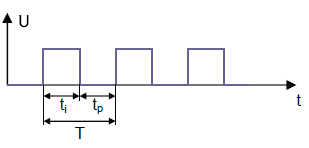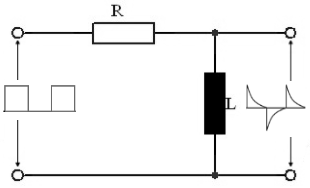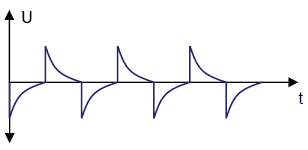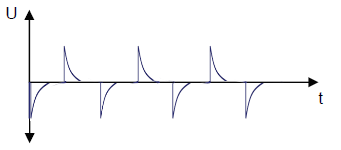# RL differentiator calculation

Calculator and formulas for calculating a RL differentiator

## RL differentiator online calculator

This function can be used to calculate the properties of a a resistor and a coil differentiating circuit. The function calculates the coil, the resistance or the period or the frequency.

Calculate differentiators

 Was soll berechnet werden ? Resistance Inductance Frequency / Period Input Resistance mΩ Ω kΩ MΩ Inductance H mH µH nH Frequency Periode Pulse Hz kHz MHz GHz pSec nSec µSec mSec Sec pSec nSec µSec mSec Sec Decimal places 0 1 2 3 4 6 8 10 Results Resistance Inductance Frequency Period

## Formulas for RL differentiator

The differentiator works as a pulse shaper. The RL element generates a pulse-like alternating voltage at the output of the circuit from a square-wave voltage at the input of the circuit.T = Period
t1 = PulseThe time constant τ (Tau) of an RL element is calculated using the following formula:

$$\displaystyle Τ= \frac{L}{R}$$    ⇒    $$\displaystyle R=\frac{L}{Τ}$$    ⇒    $$\displaystyle L= Τ · R$$

τ (Tau) is the symbol for a time constant and should not be confused with the period time T in the graphic above.

If the length of the rectangular pulse (t1) corresponds to 5 times the time constant τ of the RL element, a pulse train like the one in the figure below is created.$$\displaystyle t1= 5 · \frac{L}{R}$$    ⇒    $$\displaystyle R=\frac{5 ·L}{t1}$$    ⇒    $$\displaystyle L=\frac{t1 · R}{5}$$

If the pulse duration of the input voltage is much greater than 5 τ , short pulses equal to the input voltage are generated. The following figure shows a pulse train that occurs when the pulse length (t1) corresponds to 10 τ .$$\displaystyle t1= 10 · \frac{L}{R}$$    ⇒    $$\displaystyle R=\frac{10 ·L}{t1}$$    ⇒    $$\displaystyle L=\frac{t1 · R}{10}$$